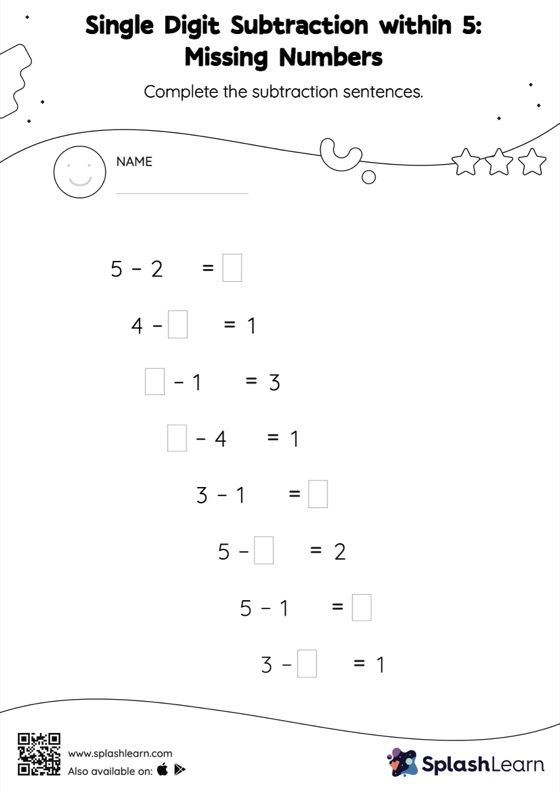# Single Digit Subtraction within 5: Missing Numbers Worksheet

Home > Single Digit Subtraction within 5: Missing NumbersChallenge your young math wizards to solve this single digit subtraction within 5 worksheet. If the missing number is a minuend or subtrahend, students use the count back strategy or the relationship between addition and subtraction to find the missing number. And if the missing number is the difference of the subtraction sentence, they subtract the numbers to get the sum. This worksheet, single digit subtraction within 5, provides plenty of practice with this concept.